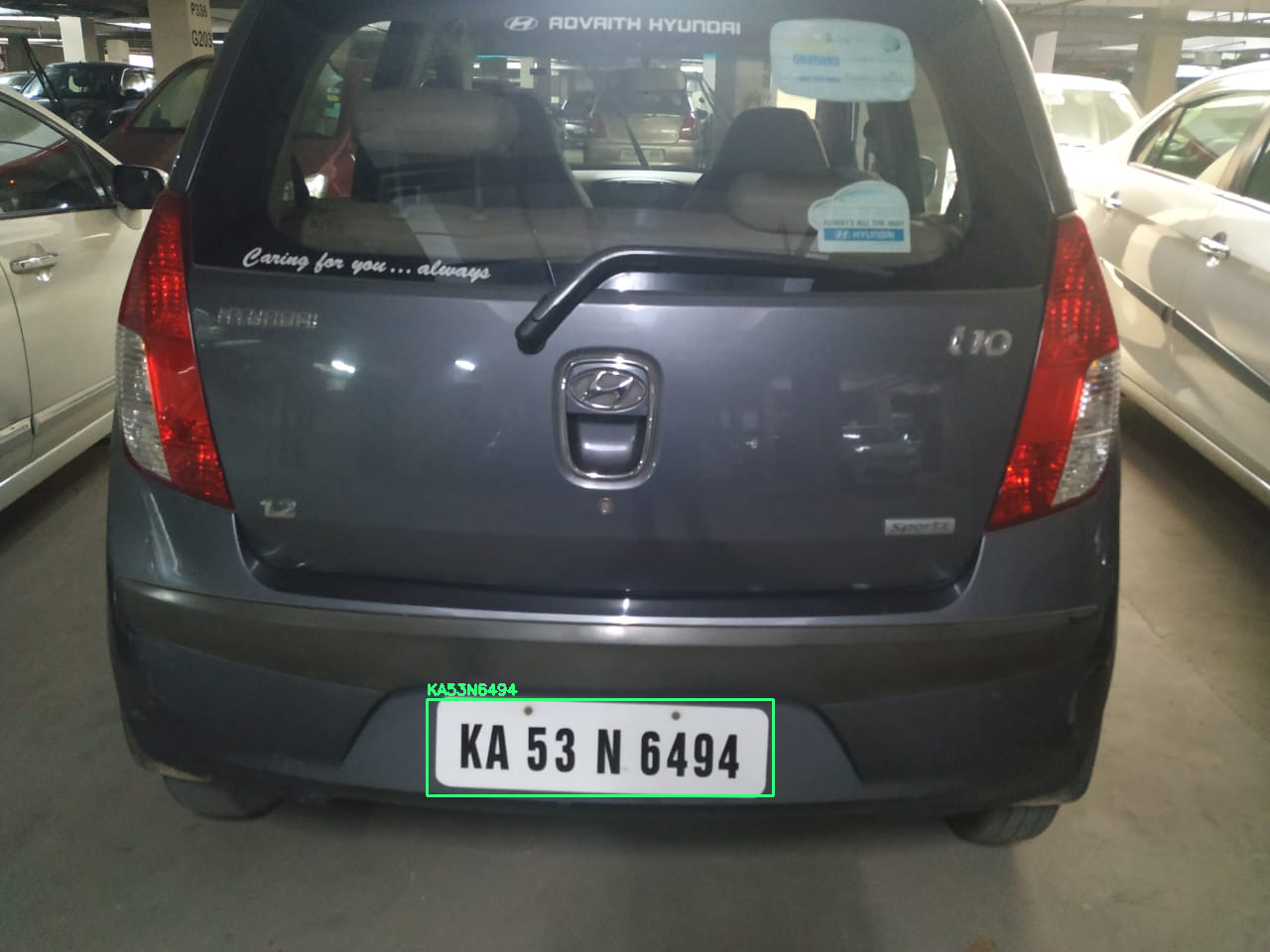# How to build a Number Plate Reader – Part 2In the previous blog of this series, we trained a model to identify a numberplate in a picture. Here we will learn how to use OpenCV and PyTesseract to get the final number from the plate.

We will start from where we ended in the last session. We had trained an ssd_inception model and used it Tensorflow Object Detection API to detect number plates.

We will first give the code for the number plate reader. The code is commented, but we will go through to explain each line.

``````import numpy as np
import tensorflow as tf
import cv2 as cv
import pytesseract
import re
import os
from os import listdir
from os.path import isfile, join
import imutils

#Change PATH names to match your file paths for the model and INPUT_FILE'
MODEL_NAME='ssd_inception_output_inference_graph_v1.pb'
PATH_TO_FROZEN_GRAPH = MODEL_NAME + '/frozen_inference_graph.pb'
INPUT_FILE='test_images/img6.jpeg'

#Read the model from the file
with tf.gfile.FastGFile(PATH_TO_FROZEN_GRAPH, 'rb') as f:
graph_def = tf.GraphDef()

def cleanup_image(img):
#Resize the image with interpolation
cv.imwrite("number_plate_original.png", img)
img = cv.resize(img, None, fx=2.5, fy=2.5, interpolation=cv.INTER_CUBIC)
cv.imwrite("number_plate_resized.png", img)
#Convert to Grey scale
img = cv.cvtColor(img, cv.COLOR_BGR2GRAY)
cv.imwrite("number_plate_greyed.png", img)

#Define the kernel for morphological operations
kernel = np.ones((3, 3), np.uint8)
# Dilation expands whitespace breaking up characters and removing noise
img = cv.dilate(img, kernel, iterations=1)
cv.imwrite("number_plate_dilated.png", img)

# Erosion makes the dark characters bigger

img = cv.erode(img, kernel, iterations=1)
cv.imwrite("number_plate_eroded.png", img)

string=(pytesseract.image_to_string(img))
string=re.sub(r'\W+', '', string)
return (string)

#Create the tensorflow session
with tf.Session() as sess:

sess.graph.as_default()
tf.import_graph_def(graph_def, name='')

rows = img.shape
cols = img.shape
inp = cv.resize(img, (300, 300))
inp = inp[:, :, [2, 1, 0]]  # BGR2RGB

# Run the model
out = sess.run([sess.graph.get_tensor_by_name('num_detections:0'),
sess.graph.get_tensor_by_name('detection_scores:0'),
sess.graph.get_tensor_by_name('detection_boxes:0'),
sess.graph.get_tensor_by_name('detection_classes:0')],
feed_dict={'image_tensor:0': inp.reshape(1, inp.shape, inp.shape, 3)})

# Visualize detected bounding boxes.
num_detections = int(out)

for i in range(num_detections):
classId = int(out[i])

score = float(out[i])
bbox = [float(v) for v in out[i]]

if score > 0.9:
# Creating a box around the detected number plate
x = int(bbox * cols)
y = int(bbox * rows)
right = int(bbox * cols)
bottom = int(bbox * rows)
#Extract the detected number plate
tmp=img[ y: bottom, x: right ]
text=cleanup_image(tmp)
text_height=0.5
cv.rectangle(img, (x, y), (right, bottom), (125, 255, 51), thickness=2)
cv.putText(img, text, (x, y - 5),
cv.FONT_HERSHEY_SIMPLEX, text_height, (125, 255, 51), 2)
``````

The first step is to create an image of only the license plate. This is done by the following code

``````        #Extract the detected number plate
tmp=img[ y: bottom, x: right ]``````

TMP is an np array that represents the detected number plate. We then send this array to a function cleanup_image.

The following steps are done in the function cleanup image.

#### upscale

We will upscale the image by 2.5 times and use basic interpolation so that detail is not lost. This makes is easier for us to manipulate the image

#### Convert To GREYSCAle

We convert the image to greyscale as it easier to delineate and read text in black and white.

#### Dilation and erosion

Dilation and Erosion are morphological operations in OpenCV. We first create a 3×3 kernel or matrix and then run a convolution over the entire image. Dilation increases the whitespace, reducing noise or small dark spots in the image. After small dark pixels are eliminated, the bigger numbers are now clearly delineated, but may be smaller. Erosion now will make the dark letters a little bigger and easier to read.

#### PyTesseract OCR

We will now use Pytesseract OCR to read the text from the image. The text in image is now quite clear for Pytesseract to read. After running Pytesseract we return the string back to the main function. The main function now makes a square around with number plate with the read vehicle number.